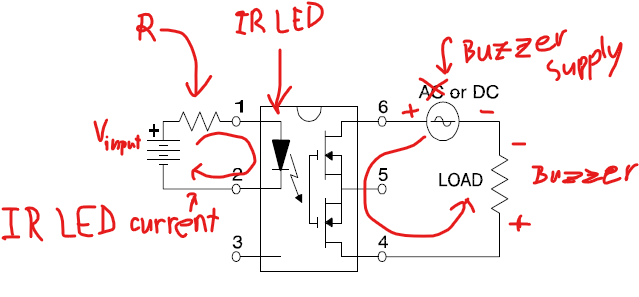# Looking for SSR

I’m trying to discourage deer from getting into my garden. To that end, I have a solar motion sensing LED floodlight. The light comes on when it senses motion; the voltage on the LED is 2.7VDC when illuminated.

I wish to add a low power sonalert to add noise to the light, but it requires higher voltage than 2.7VDC, so I’d like to use an SSR or other solid state isolation device that would use 2.7VDC as the triggering voltage and could switch 9VDC to power the sonalert.

I’ve been out of electronics for too long to figure this one out, so I need some advice on what isolating device would work.

Thanks

Ray

Hello Ray,
Welcome to the TechForum. Part 255-1567-ND is a stock part you can check for your application.

Thanks, Q.

My hesitation on all of the devices is the input voltage. Your device input is 1.16VDC where I have 2.7VDC. Will that fry the input???

Ray

Which Sonalert device were you considering? Would need to know its specs to have a good idea which SSR would be most optimal. Assuming it draws less than 500mA, here’s a short list of options to consider.

Also, devices that turn on LEDs are sometimes a bit tricky, as LEDs are current-controlled rather than voltage-controlled. This means that tapping into that signal may have unintended consequences to the LED drive circuit (reduced brightness, or possibly even not operating at all). If you measure 2.7V across the output when the LED is lit, and it is drawing more than 50mA, then putting an SSR in parallel, with proper current limiting (preferably drawing no more than 10% of the LED’s current) is not likely to significantly affect the rest of the circuit.

The typical SSR capable of being triggered by 2.7V or less uses an internal infrared (IR) LED to trigger an IR sensing MOSFET on the output side. The input IR LED needs to have a current-limiting resistor to prevent over-current of that LED, as shown here:To select the right resistor value, you need to know the input voltage of your system (presumably 2.7V, from what you state), the forward voltage drop of the SSR’s IR LED, and the required current to properly trigger that IR LED. This info can be found in the respective SSRs’ datasheets.

Once you know these, you can calculate the proper current-limiting resistor value by figuring out the required voltage across it and the current to be passed through it. The voltage across the resistor can be calculated by subtracting the IR LED forward voltage from your input voltage (presumably 2.7V in this case). Since the resistor will be in series with the IR LED, the current passing through the resistor will be the same as that passing through the IR LED.

Then using Ohm’s Law, since resistance can be calculated by dividing the voltage across the resistor by the current passing through it, you get the following equation:

Rcurrent limiting resistor = (Vinput - VIRLED) / IIR LED current

Since the current passing through the IR LED is typically set to 10mA or less, the power rating of the resistor is typically not a worry. Therefore, 1/4 Watt or 1/8 Watt should suffice.

Thanks, Q.

My hesitation on all of the devices is the input voltage. Your device input is 1.16VDC where I have 2.7VDC. Will that fry the input???

Ray

Hello Ray,

According to the Datasheet for 255-1567-ND, the maximum LED reverse voltage is 5V. So it should handle 2.7VDC. The best option with a higher input rating, is TLP3553A(F-ND, which is rated for 1.64VDC input. The maximum input reverse voltage of this one, is listed as 6V, on the Datasheet.

Hi Ray,

See my schematic and explanation above. A series resistor is required to operate these devices. The resistor both limits the current and drops the excess voltage down to the proper level for the input LED of the SSR. This is what protects the input IR LED. So, for the 255-1567-ND mentioned above (Panasonic part number AQZ102), for example, in the datasheet we see that the forward voltage drop will be approximately 1.16V when driven with 10mA. This will vary with temperature, but since 10mA is well below the maximum and well above the minimum LED current, it is an optimal value to shoot for.

Using the formula I gave you above, you would calculate the proper resistor value for current limiting as follows:

Rcurrent limiting resistor = (Vinput - VIRLED) / IIR LED current

Rcurrent limiting resistor = (2.7V - 1.16V) / 0.01A = 154 Ohms

The nearest common value resistor is 150 Ohms, so that would be the value to use. Here’s a link to several which would work.

1 Like

I want to thank you for all the info you’ve provided, and for the time you spent in sharing it. It is much appreciated. I’ll be ordering the SSR and resistor later today.

1 Like